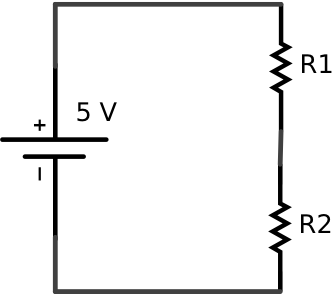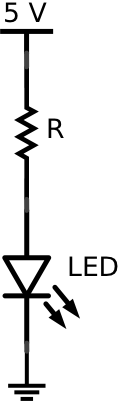by

# The Voltage Divider Circuit and LED Current Limiting

### Ohm’s Law

Ohm’s Law is a mathematical relationship between the current flowing through, voltage supplied to, and the resistance of an electric circuit given by,

`V=IR`

where `V` is voltage in volts (V), `I` is current in amperes (A), and `R` is resistance in ohms (Ω). Using the analog/digital trainer, build the following circuit using a 1 kΩ resistor (`R1`) and a 1.5 kΩ resistor (`R2`) connected in series.A) Use a digital multimeter to measure the current flowing through and the voltage drop across each resistor.

B) Note that when two resistors are connected in series the total resistance of the circuit is the sum of the individual resistors (`R=R1+R2`). Use this fact and Ohm’s Law to calculate the theoretical current flowing through the circuit. How does your actual measurement compare to this theoretical value (calculate a %error).

C) Once the current is known, use Ohm’s Law applied to each resistor individually to calculate the voltage “used” by each resistor. How do your actual measurements compare to these theoretical values (calculate %errors).

### The Voltage Divider Circuit

The circuit you constructed above is often referred to as a voltage divider circuit because the total voltage supplied to the circuit is “divided” up or shared by the resistors. The values of the resistors determine the division of the voltage.

D) Use Ohm’s Law to derive an expression for the voltage across `R2` as a function of `V`, `R1`, and `R2`. This expression gives you a tool to design a circuit to provide any conceivable voltage by taking an output across `R2`. Importantly, whatever circuit is connected to the voltage divider circuit can not draw current or the properties of the circuit will be altered.

### Using a Resistor to Limit the Current to an LED

A red LED typically has a forward bias voltage of 1.8 to 2.2 V and a recommended forward current of 16 to 20 mA. Let’s assume you have a red LED in your possession that requires a forward bias voltage to 2 V to turn on and draws 20 mA at that voltage. If you want to safely power such an LED with a 5 V power supply then you should use a voltage divider circuit where the LED takes the place of `R2` and the value of the other resistor is selected so the voltage drop across the LED is 2 V and the current flowing through the circuit is 20 mA. The figure below shows such a circuit with an LED and a current-limiting resistor in series.E) Use Ohm’s Law and the voltage divider formula derived above to calculate the resistor `R` value that limits the current in the circuit to 20 mA and the voltage across the LED to 2 V.
F) Build and test this circuit.